1 2 3 ... 29 30 »

# Ball Rolling on Tilted Turntable

Michael Fowler

## Introduction

We'll now consider an interesting dynamics problem not covered in most introductory texts, a rolling ball on a rotating, possibly tilted, surface. As we'll see, this tough-sounding problem is not that difficult to solve using Newtonian methods, and leads to some surprising results. For example, a ball rolling on a steadily rotating horizontal plane moves in a circle, and not a circle centered at the axis of rotation. We'll prove this -- and demonstrate it in class. Even more remarkably, if the rotating plane is tilted, the ball follows a cycloidal path, keeping at the same average height -- not rolling downhill. (This is exactly analogous to an electron in crossed electric and magnetic fields!) One reason the rolling ball problems are generally avoided is that they do not readily lend themselves to a Lagrangian analysis, but can in fact be solved quite quickly with a vectorized application of Newton's laws. The appropriate techniques are described in Milne's book Vectorial Mechanics, which we follow.

## Holonomic Constraints and non-Holonomic Constraints

A spherical ball rolling on a plane without slipping is constrained in its translational and rotational motion by the requirement that the point of the sphere momentarily in contact with the plane is at rest. How do we incorporate this conditi ...

Category: Education | Views: 1201 | Added by: farrel | Date: 2017-09-01 | Comments (0)

# Motion in a Non-inertial Frame of Reference

Michael Fowler

## The Lagrangian in Accelerating and Rotating Frames

This section concerns the motion of a single particle in some potentialin a non-inertial frame of reference. (We'll userather thanfor potential in this section, since we'll be using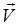for relative frame velocity.) The most general noninertial frame has both linear acceleration and rotation, and the angular velocity of rotation may itself be changing.

Our strategy is to begin with an inertial framethen go to a frame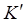having linear acceleration relative to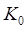then finally t ...

Category: Education | Views: 1094 | Added by: farrel | Date: 2017-09-01 | Comments (0)

# Euler's Equations

Michael Fowler

## Introduction

We've just seen that by specifying the rotational direction and the angular phase of a rotating body using Euler's angles, we can write the Lagrangian in terms of those angles and their derivatives, and then derive equations of motion. These can be solved to describe precession, nutation, etc.

One might hope for a more direct Newtonian approach -- we know, for example, that the steadily precessing child's top is easy to understand in terms of the gravitational torque rotating the angular momentum vector. What about applying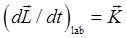(the external torque on the system) more generally? It's certainly valid. The problem is that in the lab frame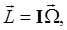meaning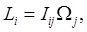and the elements of the inertia tensor relative to the lab axes are constantly changing as the body rotates. The Newtonian approach is only practicable if the connection betweencan be made in the body frame defined by ...

Category: Education | Views: 1130 | Added by: farrel | Date: 2017-09-01 | Comments (0)

# Euler's Angles

Michael Fowler

## Introduction

So far, our analysis of rotational motion has been of essentially one dimensional, or more precisely one angular parameter, motion: rotating about an axis, rolling, precessing, etc. But this leaves out many interesting phenomena: the wobbling of a slowing down top, nutation, and so on. We need a well-defined set of parameters for the orientation of a rigid body in space to make further progress in analyzing the dynamics.

The standard set is Euler's Angles. What you actually observe as you watch a child's top beginning to wobble as it slows down is the changing direction of the axis -- this direction is given by the first two of Euler's angles: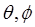which are just the usual spherical coordinates, the angle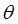measured from the vertical direction and the azimuthal angle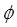about that vertical axis. Euler's third angle,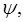specifies the orientation of the top about its own axis, completing the description of th ...

Category: Education | Views: 1136 | Added by: farrel | Date: 2017-09-01 | Comments (0)

# Rigid Body Moving Freely

Michael Fowler

## Angular Momentum and Angular Velocity

In contrast to angular velocity, the angular momentum of a body depends on the point with respect to which it is defined. For now, we take it (following Landau, of course) as relative to the center of mass, but we denote it by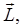following modern usage. This "intrinsic" angular momentum is like the Earth's angular momentum from its diurnal rotation, as distinct from its orbital angular momentum in going around the Sun.

That is,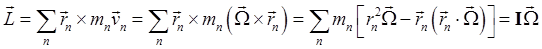where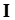is the inertia tensor: this just means.

Explicitly, taking the principal axes as the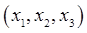axes,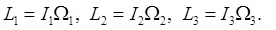For anything with spherical inertial symmetry ...

Category: Education | Views: 1133 | Added by: farrel | Date: 2017-09-01 | Comments (0)